# Involutes Curve Engineering Drawing

An involute is a curve traced by a point on a perfectly flexible string, while unwinding from around a circle or polygon the string being kept taut (tight). It is also a curve traced by a point on a straight line while the line is rolling around a circle or polygon without slipping.

To draw an involute of a given square.

Construction

1. Draw the given square ABCD of side a.
2. Taking A as the starting point, with centre B and radius BA=a, draw an arc to intersect the line CB produced at P1.
3. With Centre C and radius CP1 =2 a, draw on arc to intersect the line DC produced at P2.
4. Similarly, locate the points P3 and P4.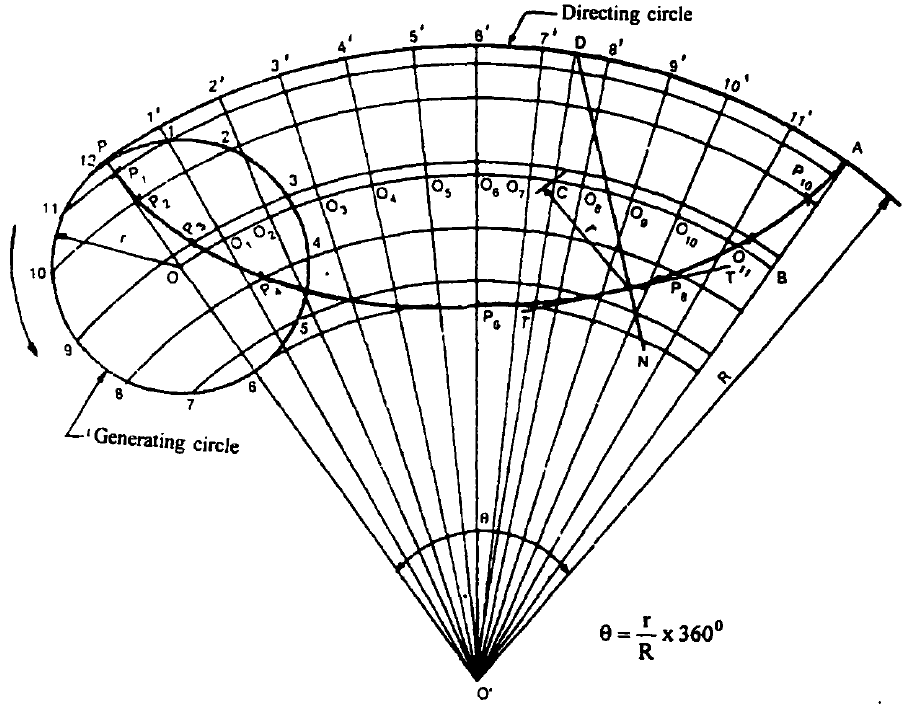Fig. 3 Construction of a Hypo-Cycloid

The curve through A, P1‘ P2, P3 and P4 is the required involute.

A P4 is equal to the perimeter of the square.

Note: To draw a normal and tangent to the curve at any point, say M on it, as M lies on the arc P3 P4 with its centre at A, the line AMN is the normal and the line TT drawn through M and perpendicular to MA is the tangent to the curve.

Involutes of a triangle, Pentagon and Hexagon are shown Figs. 5 to 7

To draw an involute of a given circle of radus R.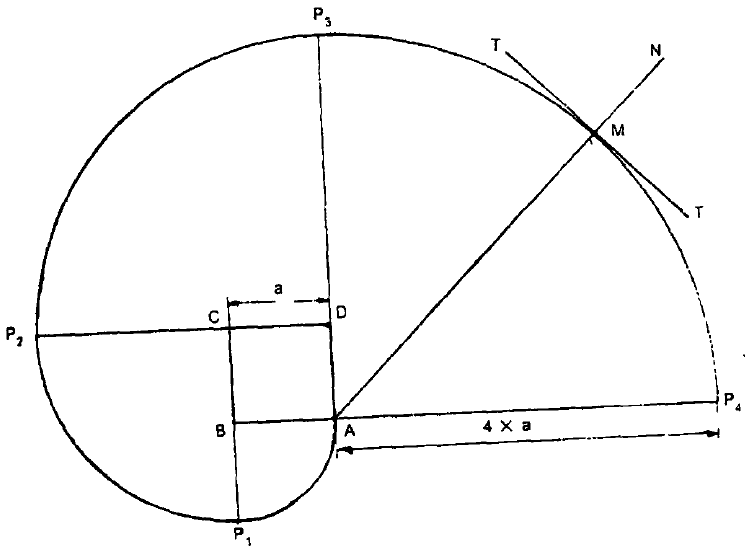Fig. 4

Construction

1. With 0 as centre and radius R, draw the given circle.
2. Taking P as the starting point, draw the tangent PA equal in length to the circumference of the circle.
3. Divide the line PA and the circle into the same number of equal pats and number the points.
4. Draw tangents to the circle at the points 1,2,3 etc., and locate the points P1‘ P2, P3 etc., such that IP1 = P11, 2P2 = P21 etc.

A smooth curve through the points P, P1‘ P2 etc., is the required involute.

Note:

1. The tangent to the circle is a normal to the involute. Hence, to draw a normal and tangent at a point M on it, first draw the tangent BMN to the circle. This is the normal to the curve and a line IT drawn through M and perpendicular to BM is the tangent to the curve.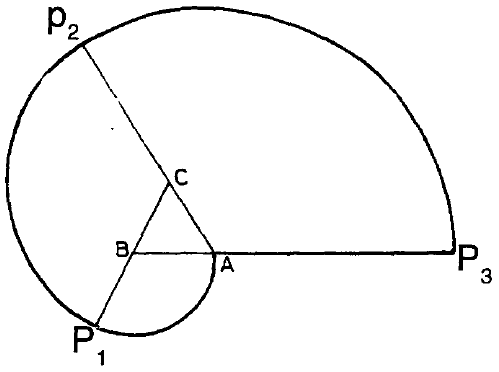Fig. 5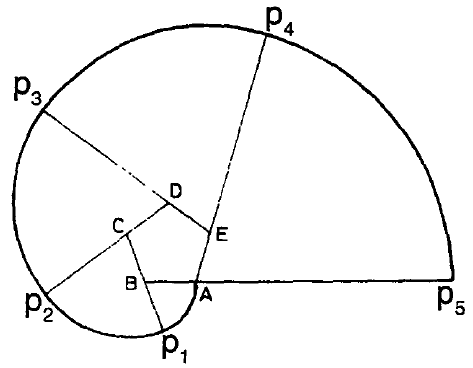Fig. 6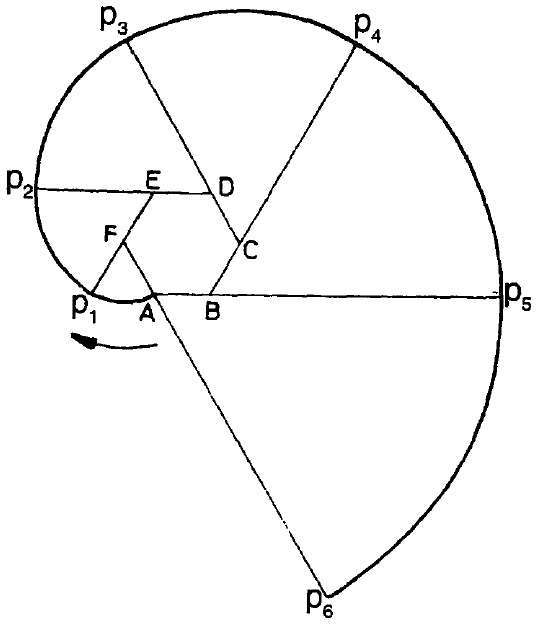Fig. 7

2. The gear tooth profile is nonnally of the involute curve of circle as shown in Fig 9.

EXAMPLES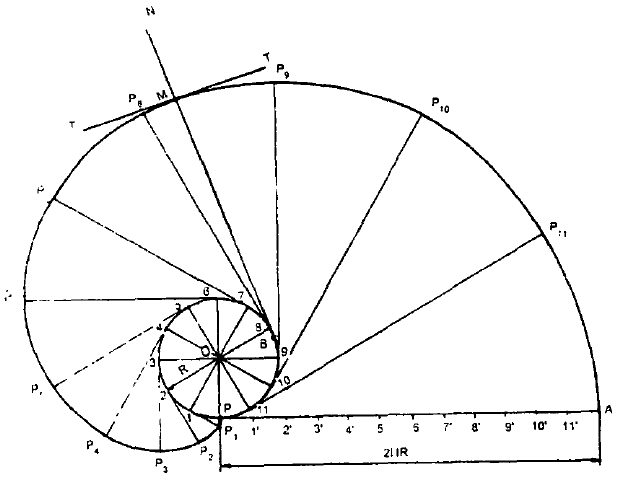Fig. 8

Problem: Construct a conic when the distance of its focus from its directrix is equal to 50 mm and its eccentricity is 2/3. Name the curve, mark its major axis and minor axis. Draw a tangent at any point, P on the curve.

Solution: (Fig. 10)

1. As the eccentricity is less than 1, the curve is an ellipse.
2. Draw one directrix, DD and the axis, AA1 perpendicular to DD and mark the focus, F such that FA = 50 mm.
3. As the eccentricity is 2/3, divide FA into 2 + 3 = 5 equal parts. Bydefmition VFNA=2/3 and hence locate the vertex, V. Draw VE perpendicular to the axis such that VE = VF. Join AE and extend it as shown in Fig. 4.50. This is the eccentricity scale, which gives the distances directly in the required ratio. In triangle AVE, VE/VA=VF/VA=2/3
4. Mark any point 1 on the axis and draw a perpendicular through it to intersectAE produced at 11. With centre F and radius equal to 1-11 draw arcs to intersect the perpendicular through 1 at P1 both above and below the axis of the conic.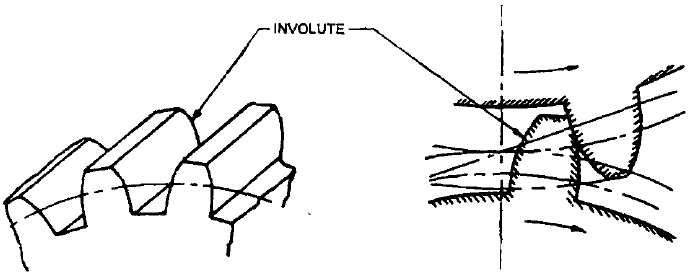Fig. 9 Involute of a circle as gear tooth profile

5. Similarly, mark points 2, 3, 4, etc., as described above.
6. Draw a smooth curve passing through the points V, P1‘ P2‘ etc., which is the required ellipse.
7. Mark the centre, C of the ellipse and draw a perpendicular GH to the axis. Also mark the other focus P such that CF1 = CP.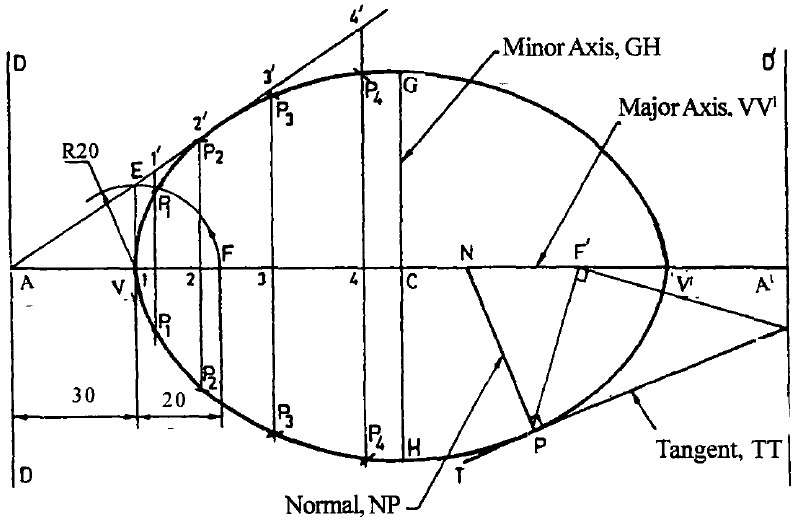Fig. 10 Construction of an Ellipse (given focus and directrix) D1

8. Tangent at any point P on the ellipse can be drawn, by joining PF1 and by drawing F1T perpendicular to PF1. Join TP and extend. Draw NP perpendicular to TP. Now, TPT and NPN are the required tangent and normal at P respectively.

Problem: The foci of an ellipse are 90 mm apart and the major axis is 120 mm long. Draw the ellipse by using four centre method.

Solution: (Fig. 11)

1. Draw the major axis AB = 120 mm. Draw a perpendicular bisector COD. Mark the foci F and P such that FO = F1O = 45 mm.
2. With centre F and radius = AO = 60 mm draw arcs to cut the line COD at C and D as shown in Fig. 11. Now, CD is the minor axis.
3. Join AC .With O as centre and radius = OC draw an arc to intersect the line AB at E.
4. With C as centre and AE as radius draw an arc to intersect the line AC at G
5. Draw a perpendicular bisecator of the line AG to intersect the a.xis AB at O1 and the axis CD (extended) at O2‘ Now O1 and O2 are the centres of the two arcs. The other two centres O3 and O4 can be located by taking OO3 = OO1 and OO4 = OO2‘ Also locate the points 1,2,3 and 4 as shown.
6. With centre O3 and radius = O3B draw an arc 4B3 and with centre O2 and radius = O2C draw an arc 1C4.
7. Similarly draw arcs for the remaining portion and complete the ellipse.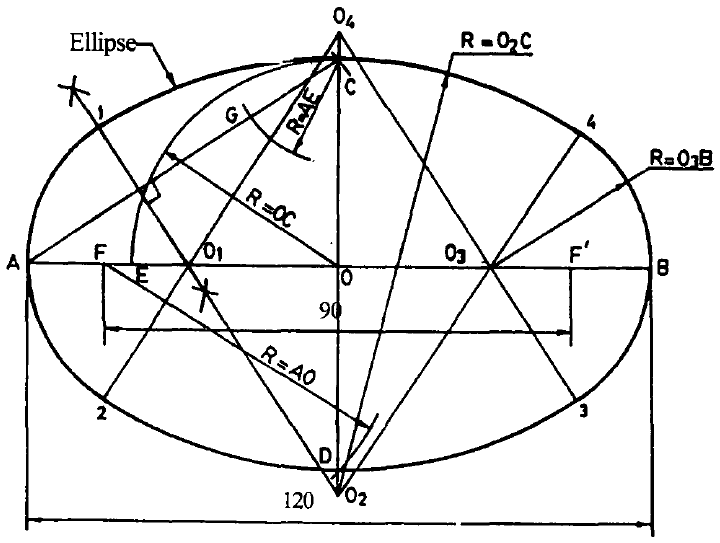Fig. 11 Construction of an Ellipse (four-centre method)

Problem : Construct an ellipse when its major axis is 120mm and minor axis is 80mm.

Solution: (Fig. 12)

1. Take a strip of paper (Tramel) and mark PQ = half the minor a.xis and PR = half the major as shown.
2. Draw AB = 120 mm to liepresent the major axis and bisect it at O. Through O draw a vertical CD = 80 mm to represent the minor axis.
3. Keep the trammel such that Q is lying on the major axis and R on the minor xis. Now the position of the point P is one of the points on the ellipse.
4. Then change the position of the trammel such that Q and R always lie on AB and CD respectively. Now the new position of the pint P is another point to construct the ellipse.
5. Repeat the above and rotate the trammel for 360°, always keeping Q along AB and R along CD.
6. For different positions of Q and R, locate the positions of point P and draw a smooth ellipse.

Problem: Construct an ellipse when its major axis is 90 mm and minor axis is 55 mm.

Solution : (Fig. 13)

1. Draw the major axis AB = 90 mm and bisect it at O. Through O draw a vertical line CD=55mm.
2. To represent diameters draw two concentric circles.
3. Divide the circles into 12 number of equal parts and draw the radial lines. These radial lines intersect the major and minor axes circles at 1,2, … 12 and 11,21, ••• .121 respectively.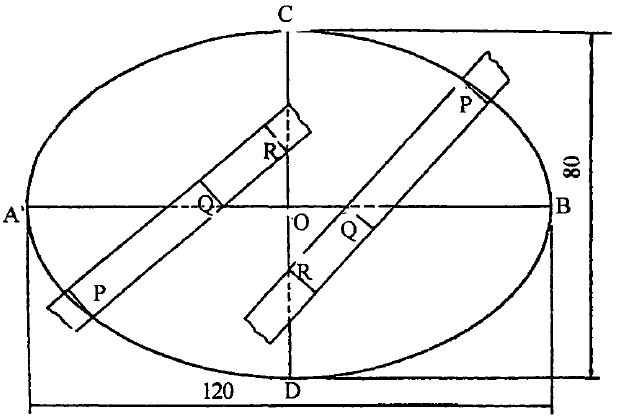Fig. 12 Trammel Method

4. From 1 draw a vertical line (parallel to CD) and frOID 11 draw a horizontal line (parallel to AB). Both intersect at P1.
5. Repeat the above and obtain the points P2‘ •••• P12 corresponding to 2 and 21, … 12, and 121 respectively.
6. Draw a smooth ellipse through P1,P2,·····P12‘ P1.

Problem: A ground is in the shape of a rectangle 120 m X 60 ID. Inscribe an elliptical lawn in it to a suitable scale.

Solution : (Fig. 14)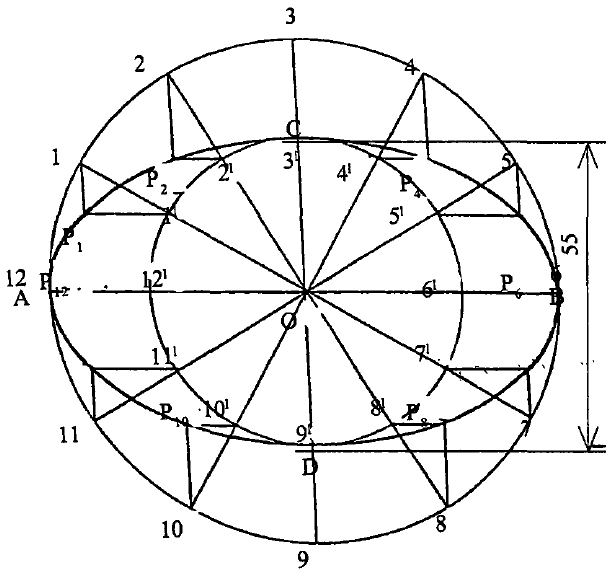Fig. 13 Concentric circles Method

1. Draw the major axis AB = 120 m and minor axis CD = 60 m. Both ILxes bisect each other at O.
2. Through A and B draw lines parallel to CD.
3. Through C and D lines parallel to AB and construct the rectangle PQRS. Now PS=AB and SR=CD.
4. Divide AQ and AP into any number of equal parts (4 say) and name the points as 1,2,3 and 11 21 31 respectively starting from A on AQ and AP.
5. DivideAO into same number of equal parts, and name the points as 11,21,31 starting from A on AO.
6. Join 1,2,3 with C. Join D11 and extend it to intersect at P1
7. Similarly extend D21 and D31 to intersect C2 and C3 at P2 and P3 respectively. Join 11,21,31 with D.
8. Join C11 and extend it to intersect D11, at P1.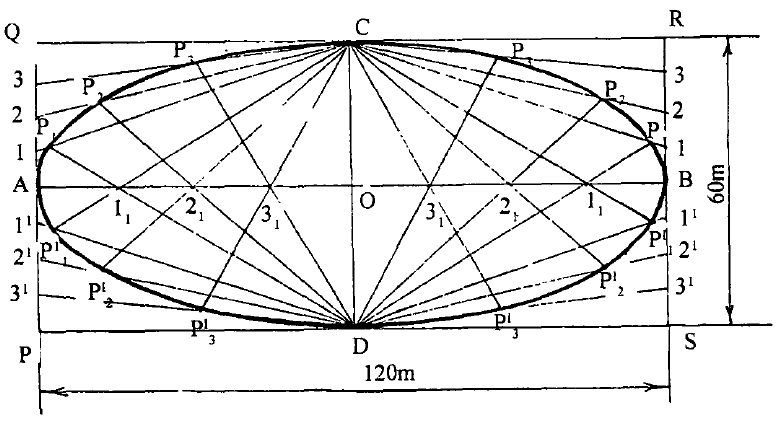Fig. 14 Rectangle (or) Oblong Method

9. Similarly extend C21 and C31 to intersect D21 and D31 at P21 and p13 respectively.
10. Draw a smooth curve through C, P3, P2, P1,A, P1, P2, P3, D and obtain one half (left-half) of the ellipse.
11. Repeat the above and draw the right-half of the ellipse, which is symmetrical to the left-half.

Problem: Construct an ellipse when a pair of conjugate diameters AB and CD are equal to 120 mm and 50 mm respecitively. The angle between the conjugate diameters is 60°.

Solution: (Fig. 15)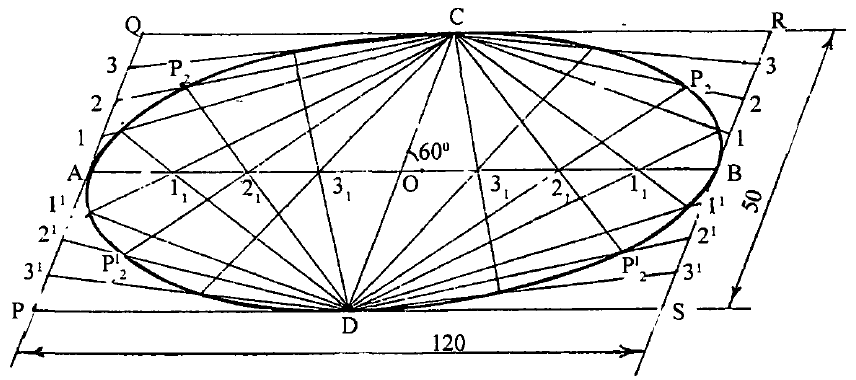Fig. 15 Parallelogram Method

1. Draw a conjugate diameter AB = 120 mm and bisect it at O.
2. Angle between the conjugte diameters is 60°. Therefore draw another conjugate diameter CD through O such that the angle COB =60°.
3. Through A and B draw lines parallel to CD. Through C and D draw lines parallel to AB and construct a parallelogram PQRS as shown in Fig. 15.
4. Repeat the procedure given in steps 4 to 10 in above problem and complete the construction of the ellipse inside the parallelogram PQRS.

Problem : Construct a conic when the distance between its focus and its directrix is equal to 60 mm and its eccentricity is one. Name the curve. Draw a tangent at any point on the curve.

Solution : (Fig. 16)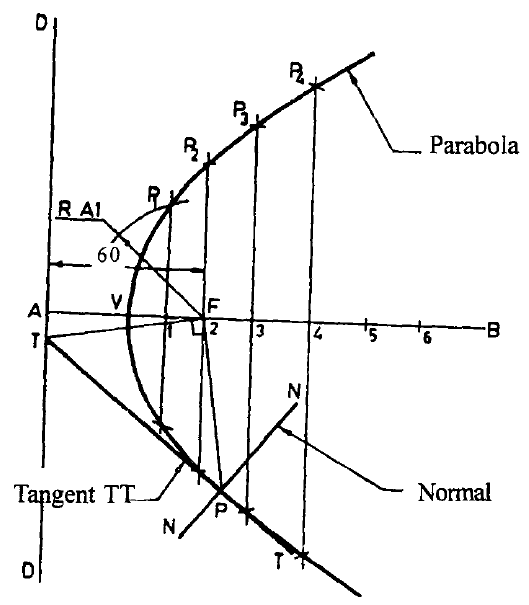Fig. 16 Construction of a Parabola

1. As the eccentricity of the conic is one, the curve is a parabola.
2. Draw the directrixDD and the axis AB perpendicular to DD. Mark the focus F such that AF = 60 mm. By definition, VF/VA = 1 and hence mark the point V, the vertex at the midpoint of AF as shown in Fig. 16.
3. Mark any number of points (say 6) on VB and draw verticals through these points.
4. With F as centre and A1 as radius draw an arc to cut the vertical through point 1 at P1. Similarly obtain points P2‘ P3, P4, etc.
5. Draw a smooth curve passing through these points to obtain the required parabola.
6. Tangent at any point P on the parabola can be drawn as follows. From point P draw the ordinate PE. With V as centre and VE as radius draw a semicircle to cut the axis produced at G. Join GP and extend it to T. Draw NP perpendicular to TP. Now, TPT and NPN are the required tangent and normal at P.

Problem : A ball thrown from the ground level reaches a maximum height of 5 m and travels a horizontal distance of 12 m from the point of projection. Trace the path of the ball.

Solution : (Fig. 17)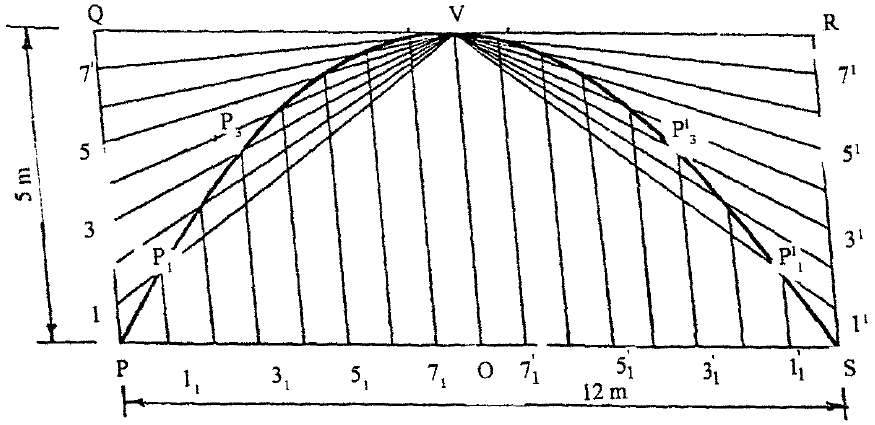Fig. 17 Rectangle Method

1. The ball travels a horizontal distance of 12 m. By taking a scale of 1: 100, draw PS = 12 cm to represent the double ordinate. Bisect PS at O.
2. The ball reaches a maximum height of 5 m. So from O erect vertical and mark the vertex V such that OV = Scm. Now OV is the abscissa.
3. Construct the rectangle PQRS such that PS is the double ordinate and PQ = RS = VO(abscissa).
4. Divide PQ and RS into any number of (say 8) equal parts as 1, 2, … 8 and 11 21 …. 81 respectively, starting from P on PQ and S on SR. Join 1,2, … 8 and 11,21 …. 81 with V.
5. Divide PO and OS into 8 equal parts as 11 21 …… 81 and 111 211….. 811 respectively, starting from P on PO and from S on SO.
6. From 11 erect vertical to meet the line V1 at P1.
7. Similarly from 21, … 81 erect verticals to meet the lines V2, …. V8 at P2 …. P2 respectively.
8. Also erect verticals from 111 211 ….. 811 to meet the lines V11 …. V21 ….. V81 at P11 …. P21 ‘” .. P81 respectively.
9. Join P, P1 P2, ••••••• P17.V1 ….. P11 and S to represent the path ofthe ball which is a parabola.

Support Us By Sharing#### Author: Aliva Tripathy

Taking out time from a housewife life and contributing to AxiBook is a passion for me. I love doing this and gets mind filled with huge satisfaction with thoughtful feedbacks from you all. Do love caring for others and love sharing knowledge more than this.